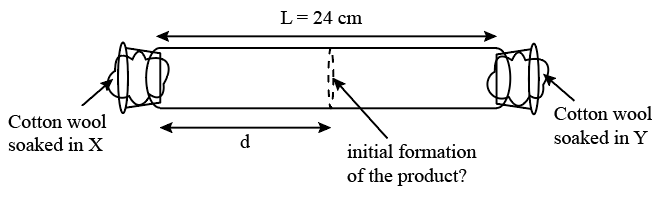### JEE (Advanced) 2014 Paper-2 Question 35

Instructions

X and Y are two volatile liquids with molar weights of $$10 g mol^{-1}$$ and $$40 g mol^{-1}$$ respectively. Two cotton plugs, one soaked in X and the other soaked in Y, are simultaneously placed at the ends of a tube of length L = 24 cm, as shown in the figure. The tube is filled with an inert gas at 1 atmosphere pressure and a temperature of 300 K. Vapours of X and Y react to form a product which is first observed at a distance d cm from the plug soaked in X. Take X and Y to have equal molecular diameters and assume ideal behaviour for the inert gas and the two vapours.Question 35